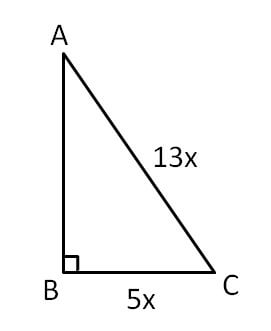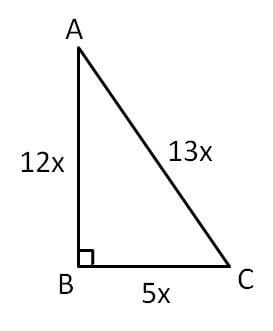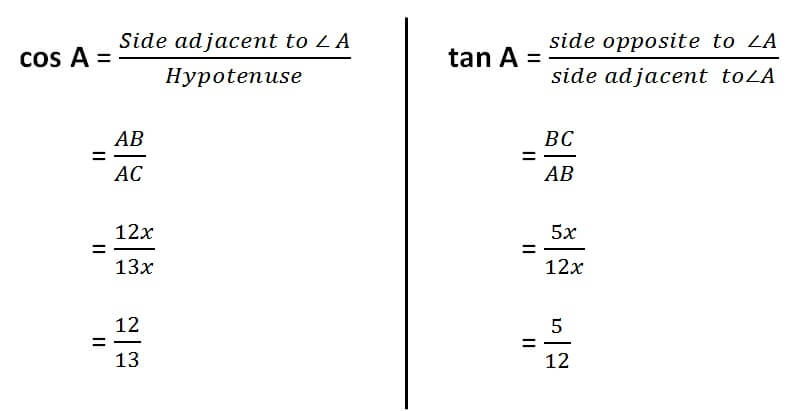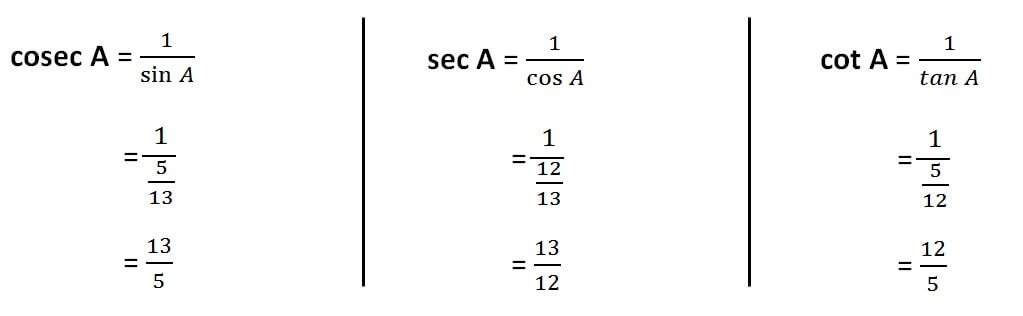Finding ratios when other ratios are given

Chapter 8 Class 10 Introduction to Trignometry
Concept wise

If sin A = 5/13. Find cos A, tan A, cosec A, sec A, cot A

sin A = 5/13

(side opposite  to  ∠A)/Hypotenuse = 5/13

⇒ BC/AC = 5/13

Let

BC = 5x

AC = 13x

We find AB using Pythagoras TheoreIn Δ ABC, using Pythagoras theorem

(Hypotenuse) 2 = (Height) 2 + (Base) 2

AC 2 = AB 2 + BC 2

(13x) 2 = AB 2 + (5x) 2

169x 2 = AB 2 + 25x 2

169x 2 – 25x 2 = AB 2

144x 2 = AB 2

AB 2 = 144x 2

AB = √144x 2

AB = √144 x

AB = 12x

So, our triangle becomesNow, we find cos A & tan ANow, let us find cosec, cot and tanLearn in your speed, with individual attention - Teachoo Maths 1-on-1 Class